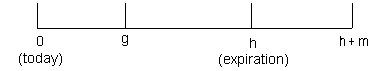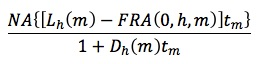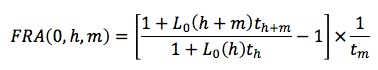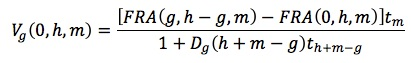### Why should I choose AnalystNotes?

Simply put: AnalystNotes offers the best value and the best product available to help you pass your exams.

##### Subject 4. Interest Rate Forward and Futures Contracts
A forward rate agreement (FRA) is a forward contract in which one party, the long, agrees to pay a fixed interest payment at a future date and receive an interest payment at a rate to be determined at expiration. It is a forward contract on an interest rate (not on a bond or a loan).

• The long pays a fixed rate and receives a floating rate. If LIBOR rises, the long will gain.
• The short pays a floating rate and receives a fixed rate. If LIBOR falls, the short will gain.

The fixed rate is also called the forward contract rate. The interest rate to be determined at expiration is also called the underlying rate.

The buyer effectively has agreed to borrow an amount of money in the future at the stated forward (contract) rate. The seller has effectively locked in a lending rate. The buyer of a FRA profits from an increase in interest rates. The seller of a FRA profits from a decline in rates.

Consider the time line shown below for an "h x (h + m)" FRA.• On day 0 we shall initiate an FRA. The FRA price is the fixed interest rate such that the FRA value is zero on the initiation date.
• The FRA expires on day h.
• The rate underlying the FRA is the rate on an m-day Eurodollar deposit.
• The date indicated by g will simply be a date during the life of the FRA at which we want to determine a value for the FRA.

Note that the market convention quotes the time periods as months, but the calculations use days based on the assumption of 30 days in a months. For example, a "1 x 3 FRA" expires in 30 days, and the payoff of the FRA is determined by 60-day LIBOR when the FRA expires in 30 days.

At time h (t1 in the following illustration), the interest rate is set and the settlement is made. This is typical for most FRAs and is referred to as "advanced set, advanced settled."A LIBOR deposit is a loan from one bank to another. Let Li(j) represent the rate on a j-day LIBOR deposit on an arbitrary day i, which falls somewhere in the above period from 0 to h. Consider a bank that borrows \$1 on day i for j days. It will pay back \$1[1 + Li(j) (j/360)] in j days.

Let's denote the fixed rate on the FRA as FRA(0, h, m), which stands for the rate on an FRA established on day 0, expiring on day h, and based on m-day LIBOR. The value at expiration (for receive-floating) is:where:

• NA = notional amount
• tm = accrual period, or m/360 (360 is the convention used in the LIBOR market)
• The numerator is the discount factor applied to the FRA payoff, because the payment is received in advance, not in arrears. Dh(m) is often assumed to be equal to Lh(m).

To find an FRA's fixed rate:This is actually the formula for a LIBOR forward rate, given the interest payment conventions in the FRA market.

• The numerator is the future value of a longer-term LIBOR of h + m days.
• The denominator is the future value of a shorter-term LIBOR of h days.
• The ratio is 1 plus a rate: subtracting 1 and multiplying by 1/tm annualizes the rate.

To value an FRA on day g:You can apply the previous FRA pricing formula to calculate FRA(g,h-g,m).

Example 2

Consider a 3 x 6 FRA, which expires in 90 days and is based on 90-day LIBOR. As we are on day 0, h = 90, m = 90.

Suppose the current rates are:

• L0(h) = L0(90) = 0.054
• L0(h + m) = L0(180) = 0.059

Using the formula: F(0, h, m) = F(0, 90, 90) = [(1 + 0.059 x 180/360)/(1 + 0.054 x 90/360) - 1] x (360/90) = 0.0631.

Therefore, to enter into an FRA on day 0, the fixed rate would be 6.31%.

Assume that we go long the FRA, and it is 20 days later. As we are on day 20, g = 20, h - g = 90 - 20 = 70, and h + m - g = 90 + 90 - 20 = 160. We also need information about the new term structure:

• Lg(h - g) = L20(70) = 0.058
• Lg(h + m - g) = L20(160) = 0.064

FRA(g, h - g, m) = FRA(20, 70, 90) = {[1+0.064 x 160/360]/[1+0.058 x 70/360] - 1} x 360/90 = 0.06790

Vg(0, h, m) = [(0.06790 - 0.0631) x 90/360]/(1 + 0.064 x 160/360) = 0.001167

Therefore, the FRA now has a value of \$0.001167 per \$1 of notional principal.

User Comment
danlan2 After interest rate contracts, there are more: fixed income contracts, currency contracts ...
marcher Jesus!!
bbadger Jesus. I think I've seen too many formulas
robertucla Dear Lord!
CIDB Dido! The only trick I've found for this is to dumb down formulas into words. I find using words instead of notation really increases my retention.
mtsimone This is clearer:

mtsimone The FRA is always based on the float. If you receive float you're long the FRA because you can't be long something that's fixed. Conversely, if you pay the float, you're short the FRA... you can't be short something that's fixed!
breh a good way to think about calculating the fixed rate is similar to the currency spot rate inferred by the forward rates